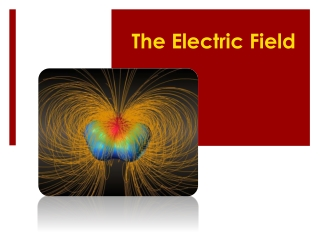DownloadDownload PresentationThe Electric Field

# The Electric Field

Download Presentation## The Electric Field

- - - - - - - - - - - - - - - - - - - - - - - - - - - E N D - - - - - - - - - - - - - - - - - - - - - - - - - - -
##### Presentation Transcript

1. The Electric Field

2. What do you think? • Electric forces and gravitational forces are both field forces. Two charged particles would feel the effects of both fields. Imagine two electrons attracting each other due to the gravitational force and repelling each other due to the electrostatic force. • Which force is greater? • Is one slightly greater or much greater than the other, or are they about the same?

3. Drawing Field Lines • In the chapter “Circular Motion and Gravitation,” you learned about the gravitational field (g). The diagram to the right shows the “g” field around Earth. • In this section, we will study the electric field “E” around charged particles. • On the next slide are three different diagrams. Make a sketch of the “E” field for each charge or combination of charges.

4. Drawing Field Lines: What do you think? • Make a sketch of the “E” field for each charge or combination of charges. • Field lines have direction • What direction do you think the field lines go around a + or – charge? 1 2 3

5. Electric Field Strength • Electric fields (E) have magnitude and direction. • The direction is defined as the direction of the force on a small, positive test charge (q0) placed in the field caused by Q. • The magnitude of the field is defined as the force per unit charge on q0.

6. Test Charges • A small test charge will not significantly affect the field • If the test charge (q0) is large, it will affect the way the charges are distributed on the charged conductor • This would change the field around the conductor. • Test charges will always be considered small enough to have no effect on the field.

7. Electric Field Strength • Combine Coulomb’s law with the definition of electric field to derive an equation for E due to a point charge. • SI unit: N/C • The field strength does not depend on the test charge.

8. Example Electric Field Strengths

9. Practice Problem Use your ninja physics skills!!! • An electric field around a charged object is 5.95  106 N/C at a distance of 0.100 m. Find the charge on the object. • Answer: 6.62  10-6 C or 6.62 C • Suppose a small test charge of 0.200 C was placed at the point that is 0.100 m from the charged object. What force would be exerted on the test charge and on the object? • Answer: 1.19 N for both test charge and object

10. Electric Field Line Rules Apply the above rules and sketch the E field around the charge shown.

11. Electric Field Line Rules Apply the above rules and sketch the E field around the charge shown.

12. Electric Field Line Rules Apply the above rules and sketch the E field around the charge shown.

13. Electric Field Lines Rules

14. Electric Field Line Rules Apply the above rules and sketch the E field around the charge combination shown.

15. Electric Field Lines Rules

16. Electrostatic Equilibrium • Electrostatic equilibrium occurs in conductors when no net motion of charges exists within the conductor. • Charges in a conductor are free to move, but are not moving when equilibrium exists. The rules below result from this fact This is because if there were an electric field inside a conductor, the free charges would move and a current would exist. This net movement of charge would mean it was not in electrostatic equilibrium. This is because if there is less space for charge distribution (and they still seek equilibrium) – thus they get more concentrated in these sharp areas This is because the charges repel each other and this forces them as far apart as possible, causing them to migrate to the surface This is because if it were not perpendicular it would have a component along the surface of the object causing the free e- to move. Thus a current would exist and there would not be electrostatic equilibrium